# Lateral Friction Loss

This is for a sprinkler lateral with sprinklers on a regular interval and is plugged at the end.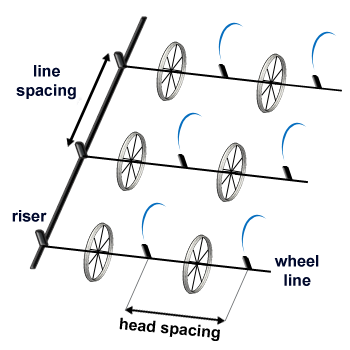Sprinklers per Line:
Sprinkler Head Spacing along the Line:
Sprinkler Flow Rate:
Pipe Size:
Pipe Type:
Total Flow Rate:
Total Pipe Length:
Friction Loss:

## The Equations

This calculator uses this formula to determine the Total Flow Rate, Total Pipe Length, and Friction Loss.

#### Total Pipe Length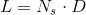Where: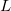= Total Pipe Length (ft)= Number of sprinklers per line= Distance between sprinklers, sprinkler spacing (ft)

#### Total Flow RateWhere: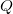= Total Flow Rate (gpm)= Sprinkler flow rate (gpm)= Number of sprinklers per line

#### Lateral Friction Loss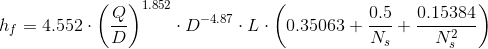Where:= Friction Loss (psi)= Total Flow Rate (gpm)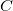= An arbitraty number to account for the pipe type (see table)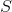= Pipe Size (in)= Total Pipe Length (ft)= Number of sprinklers per line

Pipe Type Value
Plastic 150
Aluminum 120

WSU Prosser - IAREC, 24106 N Bunn Rd, Prosser WA 99350-8694, 509-786-2226. Contact Us